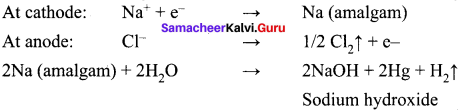# Samacheer Kalvi 11th Chemistry Solutions Chapter 5 Alkali and Alkaline Earth Metals

The Samacheer Kalvi Class 11th Chemistry Solutions Chapter 5 Alkali and Alkaline Earth Metals Questions and Answers prevailing here are designed by the academic subject expertise in accordance with the state board prescribed syllabus and books. Aspirants who require help in preparing the Tamilnadu State Board Class 11th Chemistry Solutions Chapter 5 Alkali and Alkaline Earth Metals exercise questions can rely on the Samacheer Kalvi 11th Chemistry Solutions pdf for Chapter 5 Alkali and Alkaline Earth Metals given in this article.

## Tamilnadu Samacheer Kalvi 11th Chemistry Solutions Chapter 5 Alkali and Alkaline Earth Metals

Students who are excited for Tamilnadu State Board for Class 11th Chemistry Solutions Chapter 5 Alkali and Alkaline Earth Metals can find detailed and step wise solutions for all questions of Chapter 5 Alkali and Alkaline Earth Metals from here. Simply tap on the links available over here for which you have to view/download the Samacheer Kalvi Class 11th Solutions for Chemistry Solutions chapter 5 Alkali and Alkaline Earth Metals Questions and Answers. All these solutions are free to download and easy to access online or offline so that you can prepare well for the exams at any time.

### Samacheer Kalvi 11th Chemistry Alkali and Alkaline Earth Metals Textual Evaluation Solved

Question 1.
For alkali metals, which one of the following trends are incorrect?
(a) Hydration energy : Li > Na > K > Rb
(b) Ionization energy : Li > Na > K > Rb
(c) Density : Li < Na < K < Rb
(d) Atomic size : Li < Na < K < Rb
(c) Density : Li < Na < K < Rb
Potassium is lighter than sodium. The correct order of density is
Li < K< Na < Rb < Cs
0.54 < 0.86 < 0.97< 1.53< 1.90 (in g cm3).

Question 2.
Which of the following statements are incorrect?
(a) Li+ has minimum degree of hydration among alkali metal cations.
(b) The oxidation state of K in KO2 is +1.
(c) Sodium is used to make Na/Pb alloy.
(d) MgSO4 is readily soluble in water.
(a) Li+ has minimum degree of hydration among alkali metal cations.
Li+ has maximum degree of hydration among alkali metal cations.
Li+ > Na+ > K+ > Rb+ > Cs+

Question 3.
Which of the following compounds will not evolve H2 gas on reaction with alkali metals?
(a) ethanoic acid
(b) ethanol
(c) phenol
(d) none of these
(d) none of these
Hint:
All these compounds reacts with alkali metals to evolve hydrogen gas.

Question 4.
Which of the following has the highest tendency to give the reaction Aqueous –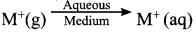(a) Na
(b) Li
(c) Rb
(d) K
(b) Li.
Hint:
Hydration energy of Li+ is more and hence Li+ is stabilized in aqueous medium.

Question 5.
Sodium is stored in ………..
(a) alcohol
(b) water
(c) kerosene
(d) none of these
(c) kerosene

Question 6.
RbO2 is ………….
(a) superoxide and paramagnetic
(b) peroxide and diamagnetic
(c) superoxide and diamagnetic
(d) peroxide and paramagnetic
(a) superoxide and paramagnetic
Hint:
RbO2 is a super oxide which contains Rb+ and O2- ions. O2- contains one unpaired electron and hence it is paramagnetic.

Question 7.
Find the wrong statement …………
(a) sodium metal is used in organic qualitative analysis
(b) sodium carbonate is soluble in water and it is used in inorganic qualitative analysis
(c) potassium carbonate can be prepared by Solvay process
(d) potassium bicarbonate is acidic salt
(c) Potassium carbonate can be prepared by Solvay process
Hint:
Potassium carbonate cannot be prepared by Solvay process. Potassium bicarbonate is fairly soluble in water and does not precipitate out.

Question 8.
Lithium shows diagonal relationship with
(a) sodium
(b) magnesium
(c) calcium
(d) aluminium
(b) magnesium (diagram pending)

Question 9.
In case of alkali metal halides, the ionic character increases in the order
(a) MF < MCl < MBr < MI
(b) MI < MBr < MCl < MF
(c) MI < MBr < MF < MCl
(d) none of these
(b) MI < MBr < MCl < MF
Hint:
Ionic character (difference in electronegativity) MI < MBr < MCl < MF

Question 10.
In which process, fused sodium hydroxide is electrolysed for extraction of sodium?
(a) Castner’s process
(b) cyanide process
(c) Down process
(d) All of these
(a) Castners process Castner’s process
NaOH ⇌ Na+ + OH
Cathode : Na+ + e → Na
Anode : 2OH → H2O + 1/2 O2 + 2e

Question 11.
The product obtained as a result of a reaction of nitrogen with CaC2 is (NEET – Phase I)
(a) Ca(CN)3
(b) CaN2
(c) Ca(CN)2
(d) Ca3N2
(c) Ca(CN)2
Hint: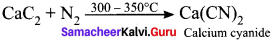Question 12.
Which of the following has highest hydration energy?
(a) MgCl2
(b) CaCl2
(c) BaCl2
(d) SrCl2
(a) MgCl2
Hint:
The order of hydration energy of alkaline earth metal is Be2+ > Mg2+ > Ca2+ > Sr2+ > Ba2+

Question 13.
Match the flame colours of the alkali and alkaline earth metal salts in the bunsen burner
(p) Sodium – (1) Brick red
(q) Calcium – (2) Yellow
(r) Barium – (3) Violet
(s) Strontium – (4) Apple green
(t) Cesium – (5) Crimson red
(u) Potassium –  (6) Blue
(a) p – 2, q – 1, r- 4, s – 5, t- 6, u – 3
(b) p – 1, q – 2, r – 4, s – 5, t – 6, u – 3
(c) p – 4, q – 1, r – 2, s – 3, t – 5, u – 6
(d) p – 6, q – 5, r – 4, s – 3, t – 1,u – 2
(a) p – 2, q – 1, r – 4, s – 5, t – 6, u – 3
(p) sodium – yellow (2)
(p) calcium – brick red (1)
(r) barium – apple green (4)
(s) strontium – crimson red (5)
(t) cesium – blue (6)
(u) potassium – violet (3)

Question 14.
Assertion : Generally alkali and alkaline earth metals form superoxides Reason : There is a single bond between O and O in superoxides.
(a) both assertion and reason are true and reason is the correct explanation of assertion
(b) both assertion and reason are true but reason is not the correct explanation of assertion
(c) assertion is true but reason is false
(d) both assertion and reason are false
(d) both assertion and reason are false
Hint:
Among alkali and alkaline earth metals, K, Rb and Cs alone forms superoxides. Superoxide O2- has 3 electron bond.

Question 15.
Assertion : BeSO4 is soluble in water while BaSO4 is not
Reason: Hydration energy decreases down the group from Be to Ba and lattice energy remains almost constant.
(a) both assertion and reason are true and reason is the correct explanation of assertion
(b) both assertion and reason are true but reason is not the correct explanation of assertion
(c) assertion is true but reason is false
(d) both assertion and reason are false
(a) both assertion and reason are true and reason is the correct explanation of assertion

Question 16.
Which is the correct sequence of solubility of carbonates of alkaline earth metals?
(a) BaCO3 > SrCO3 > CaCO3 > MgCO3
(b) MgCO3 > CaCO3 > SrCO3 > BaCO3
(c) CaCO3 > BaCO3 > SrCO3 > MgCO3
(d) BaCO3 > CaCO3 > SrCO3> MgCO3
(b) MgCO3 > CaCO3 > SrCO3 > BaCO3
Hint:
Solubility of carbonates decreases down the group.

Question 17.
In context with beryllium, which one of the following statements is incorrect?
(a) It is rendered passive by nitric acid
(b) It forms Be2C
(c) Its salts are rarely hydrolyzed
(d) Its hydride is electron deficient and polymeric
(c) Its salts are rarely hydrolyzed
Hint:
Correct statement is beryllium salts are easily hydrolyzed

Question 18.
The suspension of slaked lime in water is known as (NEET Phase – II)
(a) lime water
(b) quick lime
(c) milk of lime
(d) aqueous solution of slaked lime
(c) milk of lime
Hint:
Slaked lime Ca(OH)2. The suspension is called milk of lime and the clear solution is called lime water

Question 19.
A colourless solid substance (A) on heating evolved CO2 and also gave a white residue, soluble in water. Residue also gave CO2 when treated with dilute HCl.
(a) Na2CO3
(b) NaHCO3
(c) CaCO3
(d) Ca(HCO3)2
(b) NaHCO3
Hint: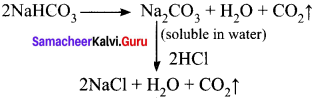Question 20.
The compound (X) on heating gives a colourless gas and a residue that is dissolved in water to obtain (B). Excess of CO2 is bubbled through aqueous solution of B, C is formed. Solid (C) on heating gives back X. (B) is ………..
(a) CaCO3
(b) Ca(OH)2
(c) Na2CO3
(d) NaHCO3
(b) Ca(OH)2
Solution: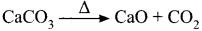CaO + H2O → Ca(OH)2
Ca(OH)2 + CO2 → CaCO3 + H2O

Question 21.
Which of the following statement is false ? (NEET – Phase -1)
(a) Ca2+ ions are not important in maintaining the regular beating of the heart
(b) Mg2+ ions are important in the green parts of the plants
(c) Mg2+ ions form a complex with ATP
(d) Ca2+ ions are important in blood clotting
(a) Ca2+ ions are not important in maintaining the regular beating of the heart
Hint:
Ca2+ ion plays an important role in maintaining regular heart beat.

Question 22.
The name ‘Blue John’ is given to which of the following compounds?
(a) CaH2
(b) CaF2
(c) Ca3(PO4)2
(d) CaO
(b) CaF2
Hint:
‘Blue john’ – CaF2 (A variety of fluorite)

Question 23.
Formula of gypsum is ………….
(a) CaSO4.2H2O
(b) CaSO4. ½2H2O
(c) 3CaSO4.H2O
(d) 2CaSO4.2H2O
(a) CaSO4.2H2O

Question 24.
When CaC2 is heated in atmospheric nitrogen in an electric furnace the compound formed is
(a) Ca(CN)2
(b) CaNCN
(c) CaC2N2
(d) CaNC2
(b) CaNCN
Solution: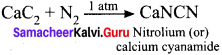Question 25.
Among the following the least thermally stable is
(a) K2CO3
(b) Na2CO3
(c) BaCO3
(d) Li2CO3
(d) Li2CO3
Hint:
Li2CO3 is least stable.

II. Write brief answer to the following questions

Question 26.
Why sodium hydroxide is much more water soluble than chloride?
NaOH + H2O ⇌ Na+ + OH
1. This reaction is an exothermic reaction. Sodium hydroxide being a strong base, completely dissociated in aqueous medium. The heat evolved increases the stability. This phenomenon is strong enough to prove that sodium hydroxide crystals are readily dissolved in water.

2. NaCl is geologically stable. If kept dry, it will remain a free flowing solid for years. Water can dissolve NaCl because the Na+ ions are attracted by OH in water and Cl ions are attracted by H+ in water. The solubility of NaCl does not increase the temperature. Based on this, NaOH is much more soluble due to exothermic nature than sodium chloride.

Question 27.
Explain what to meant by efflorescence?

1. Efflorescence is the formation of powdery deposit on the surface of rock as a result of loss of moisture or water on exposure to air.
2. Efflorescence is the formation of whitish powdery deposit on the surface of rocks like gypsum in dry regions. It is formed as mineral rich water, rises to the surface through capillary action and then evaporates.
3. Gypsum crystals are sometimes found to occur in the form that resembles the petals of flower. This happens mostly in arid areas or desert terrains, where there is rapid loss of water. This phenomenon is called as efflorescence.

Question 28.
Write the chemical equations for the reactions involved in Solvay process of preparation of sodium carbonate.
Solvay process:
The Solvay process is represented by the below chemical equations: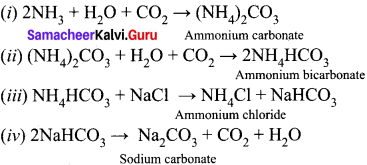Question 29.
An alkali metal (x) forms a hydrated sulphate, X2SO2. 10H2O. Is the metal more likely to he sodium (or) potassium.
X forms X2SO2. 10H2O. The metal is more likely be sodium. So X is Na2SO4. 10H2O. It is otherwise called as Glauber’s salt.

Question 30.
Write balanced chemical equation for each of the following chemical reactions.
(i) Lithium metal with nitrogen gas
(ii) Heating solid sodium bicarbonate
(iii) Rubidium with oxygen gas
(iv) Solid potassium hydroxide with CO2
(v) Heating calcium carbonate
(vi) Heating calcium with oxygen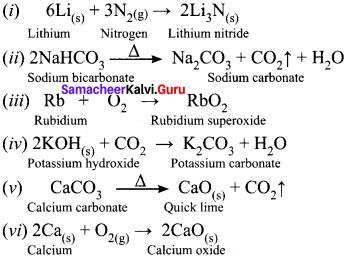Question 31.
Discuss briefly the similarities between beryllium and aluminium.
Diagonal relationship:
As observed in alkali metals, beryllium shows a diagonal relationship with aluminium. Similarities between beryllium and aluminium:

1. Beryllium and aluminium have same electronegativity values.
2. Their changes per unit area is closer.
3. BeCl2 and AlCl3 forms dimeric structure. Both are soluble in organic solvents and are strong Lewis acids.
4. Be(OH)2 and Al(OH)3 dissolves in excess alkali to give beryllate ion [Be(OH)4]2- and aluminate ion [Al(OH)4], respectively.
5. Be and Al ions have strong tendency to form complexes, e.g. BeF42- and AlF63-
6. Both Be(OFI)2 and Al(OH)3 are amphoteric in nature.
7. Carbide of Be – beryllium carbide (Be2C) and Al – aluminium carbide (Al4C3) give methane on hydrolysis.
8. Both beryllium and aluminium are rendered passive by nitric acid.

Question 32.
Give the systematic names for the following:

1. milk of magnesia
2. lye
3. lime
4. caustic potash
5. washing soda
6. soda ash and
7. trona.

1. Milk of magnesia – Mg(OH)2 – Magnesium hydroxide
2. Lye – NaOH – Sodium hydroxide
3. Lime – Ca(OH)2 Calcium hydroxide
4. Caustic potash – KOH – Potassium hydroxidc
5. Washing soda – Na2CO3. 10H2O – Sodium carbonate decahydrate
6. Soda ash – Na2CO3 – Sodium carbonate (anhydrous)
7. Trona – NaCO3.NaHCO3.2H2O – Sodium sesqui carbonate

Question 33.
Substantiate lithium fluoride has the lowest solubility among group one metal fluorides.
Lithium fluoride has high lattice enthalpy due to the small size of Li+ and F . So, due to the high lattice enthalpy, LiF is less soluble in water.

Question 34.
Mention the uses of Plaster of Paris.

• The largest use of Plaster of Paris is in the building industry as well as plasters.
• It is used for immobilizing the affected part of organ, where there is a bone fracture or sprain.
• It is also employed in dentistry, in ornamental work and for making casts of statues and busts.

Question 35.
Beryllium halides are covalent whereas magnesium halides are ionic why?
Beryllium ion (Be2+) is smaller in size and it is involved in equal sharing of electrons with halogens to form covalent bond, whereas magnesium ion (Mg2+) is bigger and it is involved in transfer of electrons to form ionic bond.

Question 36.
Alkaline earth metal (A), belongs to 3rd period reacts with oxygen and nitrogen to form compound (B) and (C) respectively. It undergo metal displacement reaction with AgNO3 solution to form compound (D).

1. An alkaline earth (A) metal belongs to third period is magnesium (Mg).
2. Magnesium reacts with oxygen to form magnesium oxide (MgO) (B).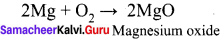3. Magnesium reacts with nitrogen to form magnesium nitride Mg3N2 (C).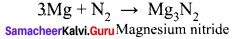4. Magnesium undergoes metal displacement reaction with AgNO3 solution to form magnesium nitrate Mg(NO3)3 (D).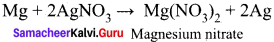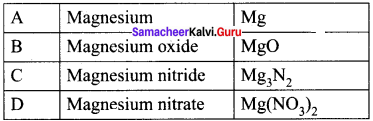Question 37.
Write balanced chemical equation for the following processes:
(a) heating calcium in oxygen
(b) heating calcium carbonate
(c) evaporating a solution of calcium hydrogen carbonate
(d) heating calcium oxide with carbon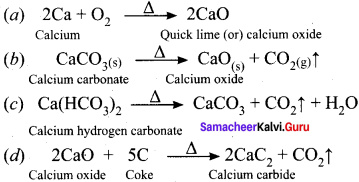Question 38.
Explain the important common features of group 2 elements. Important common features of group 2 elements.

1. Group 2 elements except beryllium are commonly known as alkaline earth metals because their oxides and hydroxides are alkaline in nature and these metal oxides are found in the Earth’s crust.
2.  Many alkaline earth metals are used in creating colours and used in fireworks.
3. Their general electronic configuration is ns2.
4. Atomic and ionic radii of alkaline earth metals are smaller than alkali metals, on moving down the group, the radii increases.
5. These elements exhibit +2 oxidation state in their compounds.
6. Alkaline earth metals have higher ionizatoin enthalpy values than alkali metals and they are less electropositive than alkali metals.
7. Hydration enthalpies of alkaline earth metals decreases as we go down the group.
8.  Electronegativity values of alkaline earth metals decrease down the group.
9. Alkaline earth metal salts moistened with concentrated hydrochloric acid gave a characteristic coloured flame, when heated on a platinum wire in a flame.

Question 39.
Discuss the similarities between beryllium and aluminium.
Diagonal relationship:
As observed in alkali metals, beryllium shows a diagonal relationship with aluminium. Similarities between beryllium and aluminium:

• Beryllium and aluminium have same electronegativity values.
• Their changes per unit area is closer.
• BeCl2 and AlCl3 forms dimeric structure. Both are soluble in organic solvents and are strong Lewis acids.
• Be(OH)2 and Al(OH)3 dissolves in excess alkali to give beryllate ion [Be(OH)4]2- and aluminate ion [Al(OH)4], respectively.
• Be and Al ions have strong tendency to form complexes, e.g. BeF42- and AlF63-
• Both Be(OFI)2 and Al(OH)3 are amphoteric in nature.
• Carbide of Be – beryllium carbide (Be2C) and Al – aluminium carbide (Al4C3) give methane on hydrolysis.
• Both beryllium and aluminium are rendered passive by nitric acid.

Question 40.
Why alkaline earth metals are harder than alkali metals?
1. The strength of metallic bond in alkaline earth metals is higher than alkali metals due to the presence of 2 electrons in its outermost shell as compared to alkali metals, which have only 1 electron in valence shell. Therefore, alkaline earth metals are harder than alkali metals.

2. The alkaline earth metals have greater nuclear charge and more valence electrons, thus metallic bonding is more effective. Due to this they are harder than alkali metals.

Question 41.
How is plaster of paris prepared?
Plaster of paris is a hemihydrate of calcium sulphate CaSO4. H2O. It is obtained by heating gypsum at 393 K.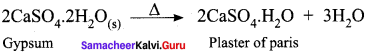Question 42.
Give the uses of gypsum.

• The Alabaster variety of gypsum was used by the sculptors.
• Gypsum is used in making dry walls or plaster boards.
• Gypsum is used in the production of Plaster of Paris, which is used as a sculpting material
• Gypsum is used in making surgical and orthopedic casts.
• It plays an important role in agriculture as a soil additive, conditioner and fertilizer
• Gypsum is used in toothpaste, shampoo and hair products.
• Calcium sulphate acts as a coagulator in making tofu.
•  It is also used in baking as a dough conditioner.
• Gypsum is a component of Portland cement, where it acts as a hardening retarder to control the speed at which concrete sets.
• Gypsum is used to give colour to cosmetics and drugs.
• Gypsum plays a very important role in wine making.

Question 43.
Describe briefly the biological importance of calcium and magnesium.

1. An adult body contains about 25 g of Mg and 1200 g of Ca. The daily requirement in the human body has been estimated to be 200-300 mg.
2. Magnesium is the co-factor of all enzymes that utilize ATP in phosphate transfer and energy release.
3. The main pigment for the absorption of light in plants is chlorophyll which contains magnesium.
4. About 99% of body calcium is present in bones and teeth.
5. Calcium plays important roles in neuromuscular function, intemeuronal transmission, cell membrane integrity and blood coagulation.
6. The calcium concentration in plasma is regulated at about 100 mgL-1. It is maintained by two hormones: calcitonin and parathyroid hormone.
7. Deficiency of magnesium results into convulsion and neuromuscular irritation.
8. 2% of adult weight is made up of calcium. Calcium phosphate is present in teeth and Calcium carbonate is present in bones. They make the teeth and bone hard.
9. Water in the human body such as inside the cell and in the blood contain dissolved calcium ions. These ions are involved in making muscles move and in sending electricity around the brain and along the nerves.
10. Magnesium is an essential element in both plant and animal life.

Question 44.
Which would you expect to have a higher melting point, magnesium oxide or magnesium fluoride? Explain your reasoning.

• Magnesium oxide has very strong ionic bonds as compared to magnesium fluoride.
• Mg2+ and O2- have charges of +2 and -2, respectively.
• Oxygen ion is smaller than fluoride ion.
• The smaller the ionic radii, the smaller the bond length in MgO and the bond is stronger than MgF2.
• Due to more strong bond nature in MgO, it has high melting point than MgF2.

### Samacheer Kalvi 11th Chemistry Alkali and Alkaline Earth Metal Additional Questions Solved

Question 1.
Which of the following is radioactive in group one elements?
(a) Lithium
(b) Caesium
(c) Rubidium
(d) Francium
(d)Francium

Question 2.
The general electronic configuration of alkali metals is ………….
(a) [noble gas] ns2
(b) [noble gas] ns1
(c) ns2 np6
(d) ns2(n-1)d1-10
(b) [noble gas] ns1

Question 3.
Among the following pairs. which occurs in large amounts in sea water?
(a) Li, Rb
(b) Cs, Fr
(c) Na, K
(d) Be, He
(c) Na, K

Question 4.
The half life period of francium is ………….
(a) 21 days
(b) 21 years
(c) 2.1 minutes
(d) 21 minutes
(d) 21 minutes

Question 5.
Spodumene and lepidolite are the minerals of …………
(a) lithium
(b) sodium
(c) potassium
(d) rubidium
(a) lithium

Question 6.
The metal present in deposits of nitre is ……………
(a) lithium
(b) potassium
(c) rubidium
(d) francium
(b) potassium

Question 7.
Rock salt is major source of ………….
(a) lithium
(b) potassium
(c) francium
(d) sodium
(d)sodium

Question 8.
Which of the following are stored under oil?
(a) Alkali metals
(b) Coinage metals
(c) Noble metals
(d) Phosphorous
(a) Alkali metals

Question 9.
The general molecular formula of rock salt or table salt is ……….
(a) NaI
(b) NaCl
(c) KCI
(d) KBr
(b) NaCl

Question 10.
The most common oxidation state of alkali metals is ………….
(a) +1
(b) +2
(c) +3
(d) +5
(a) +1

Question 11.
The second ionization enthalpy of alkali metals is
(a) zero
(b) low
(c) high
(d) very low
(c) high

Question 12.
Which of the following salt is moresoluble?
(a) NaClO4
(b) LiClO4
(c) CsBr
(d) KI
(b) LiClO4

Question 13.
Which one of the following is the smallest atom?
(a) Francium
(b) Rubidium
(c) Lithium
(d) Sodium
(c) Lithium

Question 14.
Which one of the following is less soluble in water?
(a) LiC
(b) NaCl
(c) KCl
(d) CsI
(a) LlCl

Question 15.
Consider the following statements.
(i) Alkali metals exhibit high chemical reactivity due to their low ionization energy.
(ii) Lithium is a very soft metal and even it can be cut with a knife.
(iii) Francium is a radioactive element in group 1 elements
Which of the above statements is/are not correct?
(a) (i) only
(b) (ii) only
(c) (i) and (iii)
(d) (i), (ii) and (iii)
(b) (ii) only

Question 16.
Consider the following statements.
(1) Lithium does not have d-orbitais.
(ii) Lithium carbonate is more soluble than sodium carbonate in water.
(iii),The second ionization enthalpy of alkali metals are zero.
Which of the above statements is/are not correct?
(a) (i) only
(b) (ii) only
(c) (ii) and (iii)
(d) (i), (ii) and (iii)
(c) (ii) and (iii)

Question 17.
Which one of the following gives red colour in flame test?
(a) Lithium
(b) Sodium
(c) Potassium
(d) Francium
(a) Lithium

Question 18.
Which colour is produced when alkali metals dissolved in liquid ammonia?
(a) Red
(b) Green
(c) Blue
(d) Violet
(c) Blue

Question 19.
Consider the following statements.
(i) Superoxides of alkali metals are diamagnetic.
(ii) Superoxides of alkali metals are blue in colour.
(iii) Superoxides of alkali metals are paramagnetic.
Which of the above statements is/are not correct?
(a) (i) only
(b) (ii) only
(c) (iii) only
(d) (i) and (ii)
(d) (i) and (ii)

Question 20.
Which one of the following alkali metals is highly reactive with water?
(a) Li
(b) Cs
(c) Rb
(d) K
(d) K

Question 21.
The colour produced by potassium when burnt in Bunsen flame is …………
(a) red
(b) blue
(c) green
(d) lilac
(d) lilac

Question 22.
Which of the following is insoluble in water?
(a) LiF
(b) NaCl
(c) KBr
(d) Li
(d) Li

Question 23.
Which of the following ions perform important biological functions in maintenance of the ion balance and nerve impulse conduction?
(a) Li+, Rb+
(b) Na+, K+
(c) Cs+,Fr+
(d) Rb+, Cs+
(b) Na+, K+

Question 24.
Which is the function of sodium . – potassium pump?
(a) Maintenance of ion balance
(b) Used in nerve impulse conduction
(c) Transmitting nerve signals
(d) Regulates the blood level
(c) Transmitting nerve signals

Question 25.
Which of the following ions arc more responsible for transmission of nerve signal?
(a) Li+
(b) Rb+
(c) Cs+
(d) K+
(d) K+

Question 26.
Which of the following fruits contain maximum of potassium?
(a) Grapes
(b) Potatoes
(c) Bananas
(d) Mangoes
(c) Bananas

Question 27.
Among the alkaline earth metals, which is radioactive in nature?
(a) Beryllium
(b) Calcium
(d) Barium

Question 28.
Among the following, which is the fifth most abundant element?
(a) Beryllium
(b) Barium
(d) Calcium
(d) Calcium

Question 29.
Celestite and strontianite are the ores of ………..
(a) cesium
(b) strontium
(c) magnesium
(d) barium
(b) strontium

Question 30.
The eighth most abundant among the alkaline earth metals is ………….
(a) Cu
(b) Be
(c) Mg
(d) Ca
(c) Mg

Question 31.
Which one of the following gives green spark in fire works?
(a) Magnesium chloride
(b) Sodium chloride
(c) Barium bromide
(d) Potassium iodide
(a) Magnesium chloride

Question 32.
The correct electronic configuration of alkaline earth metal is …………
(a) [noble gases] ns1
(b) [noble gases] ns2 np6
(c) [noble gases] ns2
(d) ns2 (n-1)d1-10
(c) [noble gases] ns2

Question 33.
Copper chloride produces colour in fire works.
(a) red
(b) green
(c) blue
(d) yellow
(c) blue

Question 34.
In fire works red colour flash is produced by ………….
(a) Ba
(b) Ra
(c) Sr
(d) Rb
(c) Sr

Question 35.
The most common oxidation state of alkaline earth metals is ……………
(a) +4
(b) +2
(c) + 1
(d) +3
(b) +2

Question 36.
Consider the following statements.
(i) Beryllium has small size and high polarizing power.
(ii) Beryllium does not have d-orbitais in its valence shell.
(iii) Beryllium has low electronegativity and very low ionization enthalpy as compared to other alkaline earth metals.
Which of the above statements is/are not correct?
(a) (i) only
(b) (ii) only
(c) (iii) only
(d) (i) and (ii)
(c) (iii) only

Question 37.
Hydroxides of beryllium are in nature.
(a) neutral
(b) basic
(c) acidic
(d) amphoteric
(d) amphoteric

Question 38.
When beryllium carbide reacts with water, the product mainly formed is
(a) çthane
(b) methane
(c) acetylene
(d) ethene
(b) methane

Question 39.
Which one of the following alkaline earth metal is not readily attacked by acids?
(a) Magnesium
(b) Calcium
(c) Beryllium
(d) Strontium
(c) Beryllium

Question 40.
Which metal is used as radiation windows for X-ray tubes?
(a) Magnesium
(b) Sodium
(c) Calcium
(d) Beryllium
(d) Beryllium

Question 41.
Which one of the following is used to build the beam pipe in accelerators?
(a) Be
(b) Ca
(c) Mg
(d) Sr
(a) Be

Question 42.
Which one of the following is used as a desulphurismg agent for iron and steel?
(a) Cd
(b) Mg
(c) Zn
(d) Be
(b) Mg

Question 43.
Which metal is used in photoengrave plates in printing industry?
(a) Co
(b) Pt
(c) Zn
(d) Mg
(d) Mg

Question 44.
Which can be used in controlling galvanic corrosion?
(a) Iron
(b) Magnesium
(c) Calcium
(d) Beryllium
(c) Calcium

Question 45.
Which is used in dehydrating oils?
(a) Calcium
(b) Magnesium
(c) Beryllium
(a) Calcium

Question 46.
Correctly match the list-I and list-II using the code given below the list.
List-I
A. Beryllium
B. Calcium
C. Magnesium
D. Barium

List-II
1. Sacrificial anode
3. Scavenger to remove oxygen in TV
4. Getter in vacuum tubes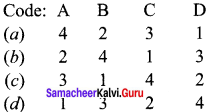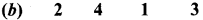Question 47.
Correctly match the list-I and list-II using the code given below the list.
List-I
A. Beryllium
B. Magnesium
C. Calcium
D. Strontium

List-II
1. Cement
2. Dating of rocks
3. X-ray detector
4. Missile construction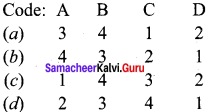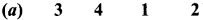Question 48.
Correctly match the list-I and list-II using the code given below the list.
List-I
B. Barium
C. Strontium
D. Calcium

List-II
1. Dehydration of oils
2. Aircraft and watches
3. Deoxidiser in copper refining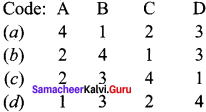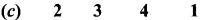Question 49.
Consider the following statements.
(i) BeO is basic.
(ii) MgO is weakly basic.
(iii) BaO is strongly acidic.
Which of the above statements is/are not correct?
(a) (i) only
(b) (ii) only
(c) (ii) and (iii)
(d) (i) and (iii)
(d) (i) and (iii)

Question 50.
Which one of the following is covalent in character?
(a) BeCl2
(b) MgCl2
(c) CaCl2
(d) BaCl2
(a) BeCl2

Question 51.
Correctly match the list-I and list-II using the code given below the list.
List-I
A. Quick lime
B. Calcium hydroxide
C. Gypsum
D. Plaster of paris

List-II
1. Casts of statues
2. Drying agent
3. White washing
4. Tooth paste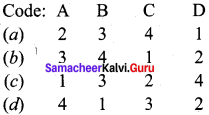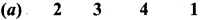Question 52.
Correctly match the list-I and list-II using the code given below the list.
List-I
A. CaO
B. Ca(OH)2
C. CaSO4 .2H2O
D. CaSO4 .½2H2O
List-II
1. Plaster of Paris
2. Quick lime
3. Slaked lime
4. Gypsum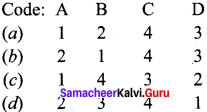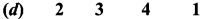Question 53.
Which one of the following is the formula of limestone?
(a) CaO
(b) Ca(OH)2
(c) CaCO3
(d) CaCO3.MgCO3
(c) CaCO3

Question 54.
Which one of the following is used in purification of sugar and as drying agent?
(a) Ca(OH)2
(b) MgSO4.7H2O
(c) CaSO4.2H2O
(d) CaO
(d) CaO

Question 55.
Which one of the following is named as bleaching powder?
(a) CaCl2
(b) CaOCl
(c) Ca(OCl)2
(d) Ca(HCO3)2
(c) Ca(OCl)2

Question 56.
Which one of the following is known as natural insulator?
(a) FeSO4.7H2O
(b) NaCO4.10H2O
(c) CaSO4.2H2O
(d) CaSO4.’/2H2O
(c) CaSO4.2H2O

Question 57.
Which one of the following is called ornamental stone?
(a) Alabaster
(b) Plaster of paris
(c) Limestone
(d) Gypsum plaster
(a) Alabaster

Question 58.
Which one of the following is used in toothpaste, shampoo and hair products?
(a) Plaster of paris
(b) Limestone
(c) Quick lime
(d) Gypsum
(d) Gypsum

Question 59.
Which one of the following plays an important role in agriculture as a soil additive, conditioner and fertilizer?
(a) Epsurn
(b) Gypsum
(c) Quick lime
(d) Salt petre
(b) Gypsum

Question 60.
Which is used to treat upset stomach and eczema?
(a) MgSO4.7H2O
(b) FeSO4.7H2O
(c) CaSO4.2H2O
(d) 2CaSO4H2O
(c) CaSO4.2H2O

Question 61.
Consider the following statements.
(i) Gypsum is used in making surgical and orthopedic casts.
(ii) Calcium nitrate acts a coagulator in making tofu.
(iii) Gypsum plays an important role in soap making.
Which of the above statements is/are not correct.
(a) (i) only
(b) (ii) and (iii)
(c) (i) and (iii)
(d) (ii) only
(b) (ii) and (iii)

Question 62.
About 393K, when Plaster of Paris is heated, it forms
(a) burnt alum
(c) gypsum plaster
(d) alabaster

Question 63.
Which of the following is used in dentistry, ornamental works and making casts of statues?
(a) CaSO4.2H2O
(b) CaSO4.½H2O
(c) CaO
(d) Ca(OH)2
(b) CaSO4.½H2O

Question 64.
Which one of the following metal act as co-factor in phosphate transfer of ATP by enzymes?
(a) Calcium
(b) Beryllium
(c) Magnesium
(d) Sodium
(c) Magnesium

Question 65.
The main pigment in plants is chlorophyll which contains ……………
(a) iron
(b) calcium
(c) barium
(d) magnesium
(d) magnesium

Question 66.
Consider the following statements.
(i) 99% of body calcium is present in bones and teeth.
(ii) The calcium concentration in plasma is regulated at 10 mg L-1.
(iii) Calcium plays an important role in neuromuscular function, interneuronal transmission and in blood coagulation.
Which of the above statements is/are not correct?
(a) (i) only
(b) (ii) only
(c) (iii) only
(d) (i), (ii) and (iii)
(b) (ii) only

Question 67.
Correctly match the list-I and list-Il using the code given below the list,
List-I
A. Chlorophyll
B. Bones
C. Dentistry
D. Cement
List-II
1. Plaster of paris
2. Gypsum
3. Magnesium
4. Calcium.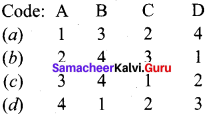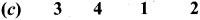Question 68.
Which one of the following is the most common alkaline earth metal found in the human body?
(a) Beryllium
(b) Magnesium
(c) Barium
(d) Calcium
(d) Calcium

Question 69.
which alkaline earth metal do not import colour to a non-luminous flame?
(a) Beryllium
(b) Calcium
(c) Magnesium
(d) Barium
(a) Beryllium

Question 70.
Statement-I: Alkali metals arc very soft metals.
Statement-II: Since the atoms of alkali metals have bigger kernels and smaller number ot valence electrons, the metallic bonds in them are very weak and hence they arc soft.
(a) Statements-I and II arc correct but statement-II is not the correct explanation of statement-I.
(b) Statements-I and II are correct and statement-II is the correct explanation of statement-I.
(c) Statement-I is correct but statement-lI is wrong.
(d) Statement-I is wrong but statement-II is correct.
(b) Statements-I and II are correct and statement-II is the correct explanation of statement-I.

Question 71.
Statement-I: BeCl2 is soluble in organic solvent.
Statement-II: Since BeCl2 is a covalent compound, it is soluble in organic solvent.
(a) Statements-I and II arc correct and statement-II is the correct explanation of statement-I.
(b) Statements-I and II are correct but statement-II is not the correct explanation of statement-I.
(c) Statement-I is wrong but statementII is correct.
(d) Statement-I is correct but statement-II is wrong.
(a) Statements-I and II are correct and statement-II is the correct explanation of statement-I.

Question 72.
Which one of the following is more basic?
(a) Ca(OH)2
(b) Mg(OH)2
(c) NaOH
(d) Al(OH)3
(c) NaOH

Question 73.
Statement-I: Cesium is considered as the most electropositive element.
Statement-II: Due to its lowest ionization energy, cesium is considered as the most electropositive element.
(a) Statements-I and II are correct and statement-II is the correct explanation of statemcnt-I.
(b) Statements-I and II are correct but statement-II is not the correct explanation of statement-I.
(c) Statement-I is correct but statement-II is wrong.
(d) Statement-I is wrong but statement-II is correct.
(a) Statements-I and II are correct and statement-II is the correct explanation of statement-I.

Question 74.
The reducing property of alkali metals follows the order
(a) Na<K<Rb<Cs<Li
(b) K<Na<Rb<Cs<Li
(c) Li<Cs<Rb<K<Na
(a) Rb<Cs<K<Na<Li
(a) Na<K<Rb<Cs<Li

Question 75.
Which of the following is the least thermally stable?
(a) MgCO3
(b) CaCO3
(c) SrCO3
(d) BeCO3
(d) BeCO3

Question 76.
Which of the following is not a peroxide?
(a) KO2
(b) CrO5
(c) Na2O2
(d) BaO2
(a) KO2

Question 77.
Which of the following is used in photoelectric cells?
(a)Na
(b) K
(e) Li
(d) Cs
(d) Cs

Question 78.
When caesìum salt is subjected to flame test, the colour produced is
(a) lilac
(b) yellow
(c) blue
(d) crimson red
(c) blue

Question 79.
Match the list-I and list-II using the correct code given below the list.
List-I
A. Lithium
B. Sodium
C. Potassium
D. Rubidium
List-II
1. Lilac
2. Reddish yiolet
3. Crimson red
4. YellowQuestion 80.
Which of the following alloy is used in making white metal bearings for motor engines?
(a) Lithium + magnesium
(c) Lithium + aluminium
(d) Lithium + copper

Question 81.
Lithium aluminium alloy is used in making
(a) armour plates
(b) white metal bearings
(c) electrochemical cell
(d) aircraft parts
(d) aircraft parts

Question 82.
Which of the following is used in making armour plates?
(a) Lithium + magnesium
(b) Lithium + aluminium
(d) Sodium + lithium
(a) Lithium + magnesium

Question 83.
Which metal is used in making electrochemical cells?
(a) Caesium
(b) Lithium
(c) Calcium
(d) Barium
(b) Lithium

Question 84.
Which of the following is used as a coolant in fast breeder nuclear reactor?
(a) Liquid ammonia
(b) Liquid helium
(c) Liquid Na metal
(d) Solid CO2
(c) Liquid Na metal

Question 85.
Which of the following is an excellent absorbent of carbon dioxide?
(a) K2 CO3
(b) CaCO3
(c) NaOH
(d) KOH
(d) KOH

Question 86.
Which of the following is used in devising photoelectric cells?
(a) Li
(b) Cs
(c) Na
(d) K
(b) Cs

Question 87.
The formula of washing soda is ……………..
(a) Na2 CO3
(b) NaHCO3
(c) Na,CO3 .10H2 O
(d) Ca(HCO3)2
(c) Na2CO3 .10H2O

Question 88.
Match the list-I and list-II using the correct code given below the list.
List-I
A. Na2CO3
B. Na2CO3 .10H2O
C. NaHCO3
D. NaOH
List-II
1. Caustic soda
2. Baking soda
3. Soda ash
4. Washing soda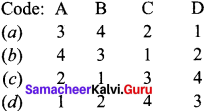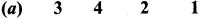Question 89.
Which one of the following is used for mercerising cotton fabrics?
(a) KOH
(b) NaOH
(c) Na2CO3
(d) NaHCO3
(b) NaOH

Question 90.
Which one of the following is used in fire extinguishers?
(a) Washing soda
(b) Soda ash
(c) Baking soda
(d) Caustic soda
(c) Baking soda

Question 91.
Assertion (A): Sodium hydrogen carbonate is used in baking cakes and pastries.
Reason (R): On heating sodium hydrogen carbonate, liberates bubbles of CO2  leaving holes in cakes and making them light and fluffy.
(a) both (A) and (R) are correct and (R) is the correct explanation of (A)
(b) both (A) and (R) are correct but (R) is not the correct explanation of(A)
(c) (A) is correct but (R) is wrong
(d) (A) is wrong but (R) is correct
(a) both (A) and (R) are correct and (R) is the correct explanation of (A)

Question 92.
Match the list-I and list-II using the correct code given below the list.
List-I
A. Manufacture of soap
B. Mild antiseptic
C. Softening of hard water
D. Coolant in nuclear reactor
List-II
1. Na2 CO3 . 10H2 O
2. Liquid Na metalli
3. NaOH
4. NaHCO3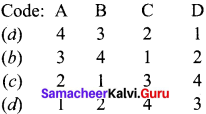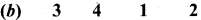Samacheer Kalvi 11th Chemistry Alkali and Alkaline Earth Metal 2-Mark Questions

Question 1.
Why alkali metals and alkaline earth metals are called s-block elements?
Alkali metals and alkaline earth metals (group 1 & 2) are those in which the last electron enters the outermost s-orbital. As the s-orbital can accommodate only two electrons, the two groups belong to the s-block elements.

Question 2.
Why group 1 elements are called alkali metals?
Group I elements form strong hydroxides on reaction with water which are strong alkaline in nature. So, group 1 elements are called alkali metals.

Question 3.
Which is the radioactive element in group 1 element? What is its half-life period?
Francium is highly radioactive element in group. It has half-life of only 21 minutes.

Question 4.
Alkali metals are stored under oil. Give reason.
Alkali metals are so reactive and they have to be stored under oil. Because when they are kept in air, they will bum immediately.

Question 5.
Name the list of elements present in alkali metal group. What is the general electronic configuration of them?
Lithium, sodium, potassium, rubidium, caesium and francium are the elements present in alkali metal group. Their general electronic configuration is [noble gas] ns1 .

Question 6.
Alkali metals never found in free state in nature. Why?
The loosely held “s” electron in the outermost shell of these elements make them as the most electropositive metals. They readily lose electron to give monovalent M ions. Hence they are never found in free state in nature.

Question 7.
The second ionization enthalpy of alkali metals are very high. Give reason.
The removal of one electron from the alkali metals causes the formation of monovalent cations having very stable electronic configuration. Therefore it becomes very difficult to remove the second electron from the stable noble gas configuration, giving rise to very high second ionization energy values.

Question 8.
LiClO4 is more soluble than NaClO4 Why?
The small size of the Li+  ion means that it has a very high enthalpy of hydration and so lithium salts are much more soluble than the salts of other group I. E.g. LiClO4  is upto 12 times more soluble than NaClO4 .

Question 9.
Why lithium has anomalous behaviour than other elements ¡n the same group?
The anomalous behaviour of lithium is due to the exceptionally small size of the atom and high polarizing power, which is a ratio of charge to radius and hydration energy.

Question 10.
Why alkali metals have high chemical reactivity? How this changes along the group?
Alkali metals exhibit high chemical reactivity because of their low ionization enthalpy and their larger size. The reactivity of alkali metals increases from Li to Cs. since the value of ionization energy decreases down the group (Li to Cs). All the alkali metals are highly reactive towards the more electronegative elements such as oxygen and halogens.

Question 11.
Lithium forms monoxide with oxygen whereas sodium forms peroxide with oxygen. Why?

• The fact that a small cation can stabilize a small anion and a large cation can stabilize a large anion explains the formation and stability of the oxides.
• The size of Li+  ion is very small and it has a strong positive field around it. It can combine with only small anion, O2- ion, resulting in the formation of monoxide Li2O.
• The Na ion is a larger cation and has a weak positive field around it and can stabilize a bigger peroxide ion, O22- or [-O-O-]2- resulting in the formation of peroxide Na2O2.

Question 12.
Explain the action of hydrogen with alkali metals.
All alkali metals react with hydrogen at about 673K (lithium at 1073K) to form their hydrides, which are ionic in nature. Reactivity of alkali metals with hydrogen increases from Li to Cs.
2M + H2 → 2 M+H (where M = Li, Na, K, Rb and Cs)

Question 13.
Alkali metal hydrides are strong reducing agents. Prove this statement.
The decrease in ionization enthalpy down the group permits easy availability of electrons to forms H ions. So, the hydrides behave as reducing agent. Their reducing nature increases down the group.

Question 14.
Explain the action of halogen with alkali metals.
Alkali metals combine readily with halogens to form ionic halides M+X. Reactivity of alkali metals with halogen increases down the group because of corresponding increase in electropositive character.
2M + X2 → 2M+X (M = Li, Na, K, Rb and Cs) (X F, Cl, Br and I)

Question 15.
Explain the action of sodium with water.
Sodium reacts so rapidly with water with the evolution of heat. The metal whizzes around the surface of water. The hydrogen gas liberated may catch fire giving yellow coloured flame
because of sodium.
2Na + 2H2O → 2NaOH + H2↑ + heat

Question 16.
What happens when potassium Is treated with water?
Potassium reacts even more violently. The liberated hydrogen catches fire instantly and burns with a lilac coloured flame because of potassium.
2K + 2H2O → 2KOH + H2↑ + heat

Question 17.
LiCl is soluble in water whereas LiBr and LiI are soluble In organic solvent. Give reason.
Lithium chloride (LiCl) is ionic in nature and it is soluble in polar solvent water, whereas lithium bromide and lithium iodide are covalent and are soluble in non-polar organic solvents.

Question 18.
Li2CO3 decomposes readily whereas other carbonates are not. Why?
The carbonates (M2CO3) of alkali metals are remarkably more stable up to 1270 K above which they first melt and then decompose to form their oxides, whereas Li2CO3 is less stable and
Li2CO3 + ∆ → Li2O + CO2
This is due to the large size difference between Li and CO32- which makes the crystal lattice unstable.

Question 19.
What are the elements present in group 2? Give their general electronic configuration.
Group 2 contains beryllium, magnesium, calcium, strontium, barium and radium. Their general
electronic configuration is [noble gas] ns2.

Question 20.
Why group 2 elements are called alkaline earth metals?
Group 2 elements except beryllium are known as alkaline earth metals. These are so called because their oxides and hydroxides are alkaline in nature and these metal oxides are found in Earth’s crust.

Question 21.
Atomic radii of alkaline earth metals are smaller than the corresponding members of alkali metals. Why?
The atomic radii of alkaline earth metals are smaller than alkali metals. This is due to the fact that group 2 elements have a higher nuclear charge, allowing the electrons to move towards the nucleus. This reduces the size of atomic and ionic radii.

Question 22.
Why beryllium has distinctive character?
The distinctive character of beryllium is mainly due to its small size, high electronegativity and high polarizing power, when compared to other elements in the same block.

Question 23.
Explain the action of halogen with alkaline earth metals.
All the alkaline earth metals combine with halogen at elevated temperature to form their halides.
M + X2 → MX2, Where M = Be, Mg,Ca,Sr,Ba and Ra. X= F,Cl,Br and I.
For e.g., Be + Cl2 → BeCl2.

Question 24.
How beryllium chloride is prepared from beryllium oxide?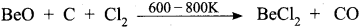Beryllium oxide is heated with carbon and chloride to get BeCl2.

Question 25.
How would you prepare beryllium hydride from beryllium chloride?
Beryllium chloride is treated with LiALH4 to get beryllium hydride.
2BeCl2 + LiAlH4 → 2BeH2 + LiCl + AlCl2

Question 26.
Mention the uses of beryllium.

• Beryllium is used as radiation windows for X-ray tubes and X-ray detectors.
• The sample holder in X-ray emission studies is made of beryllium.
• Beryllium is used to build the beam pipe in accelerators.
• Beryllium is used in detectors due to its low density and diamagnetic nature.

Question 27.
Write about the uses of strontium.

• 90Sr is used in cancer therapy.
• 87Sr / 86Sr ratio is used in marine investigators as well as in teeth, tracking animal migrations or in criminal forensics.
• Dating of rocks.
• Strontium is used as a radioactive tracer in determining the source of archaeological materials such as timbers and coins.

Question 28.

• In self-luminous paints for watches.
• In nuclear panels.
• In aircraft switches.
• In clocks and instrument dials.

Question 29.
BeO is covalent where as MgO is ionic. Give reason.
Beryllium oxide (BeO) is covalent due to the small size of Be2+ ion, while magnesium oxide (MgO) is ionic due to the bigger size of Mg2+ ion.

Question 30.
How is barium peroxide prepared?
Barium peroxide is prepared by heating monoxides with oxygen at high temperature.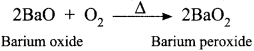Question 31.
How would you prepare quick lime?
Quick lime is produced on commercial scale by heating limestone in a lime kiln at 1173K.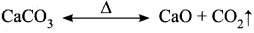This reaction being reversible, CO2 is removed as soon as it is produced to enable the reaction to proceed to completion.Question 32.
What is slaking of lime?
The addition of limited amount of water breaks the lump of lime. This process is called slaking
of lime and the product is slaked lime.
CaO + H2O → Ca(OH)2

Question 33.
What happens when quick lime reacts with –
1. H2O
2. CO2?
1. CaO + H2O → Ca(OH)2 (calcium hydroxide)
2. CaO + CO2 → CaCO3 (calcium carbonate)

Question 34.
Prove that calcium oxide is a basic oxide.
Calcium oxide is a basic oxide. It combines with acidic oxides at high temperature.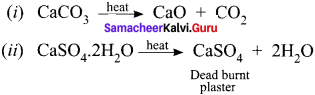Question 35.
Mention the uses of quick lime.
Calcium oxide (quick lime) is used

1. to manufacture cement, mortar and glass.
2. in the manufacture of sodium carbonate and slaked lime.
3. in the purification of sugar.
4. as drying agent.

Question 36.
What is milk of lime? How CO2 reacts with it?
The aqueous solution of calcium hydroxide is known as lime water and a suspension of slaked lime in water is known as milk of lime. When carbon dioxide is passed through lime water, it turns milky due to the formation of calcium carbonate.
Ca(OH)2 + CO2 → CaCO2 + H2O

Question 37.
What happens when excess of CO2 reacts with calcium carbonate?
CaCO3 + CO2 + H2O → Ca(HCO3)2 (Calcium bi-carbonate)

Question 38.
What is bleaching powder? How Is it prepared?
Bleaching powder is Ca(OCl)2. it is prepared by treating chlorine with milk of lime.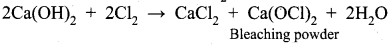Question 39.
What are the uses of calcium hydroxide?
Calcium hydroxide is used

• in the preparation of mortar, a building material.
• in white wash due to its disinfectant nature.
• in glass making and tanning industry.
• for the preparation of bleaching powder and for the purification of sugar.

Question 40.
How gypsum occurs in nature?

• Gypsum is CaSO4.2H2O. Gypsum beds were formed due to the evaporation of water from the massive prehistoric sea basins.
• When water evaporates, the minerals present in it become concentrated and crystallized.
• Gypsum is formed, due to evaporation, sulphur present in water bonds with oxygen to form a sulphate. The sulphate then combines with calcium and water to form gypsum.

Question 41.
How is gypsum synthesized?
Gypsum can also be synthesized from coal-fired power plants, as a by-product of flue-gas de suiflirization. The process of scrubbing sulfur from flue gases produced when coal is burned results in the production of several by-products, including gypsum.

Question 42.
What is meant by retrograde solubility?
Gypsum is a soft mineral and it is less soluble in water as the temperature increases. This is known as retrograde solubility, which is a distinguishing characteristic of gypsum.

Question 43.
Write a note about physical appearance of gypsum.

• Gypsum is usually white, colorless or grey in colour.
• It can also be found in the shades of pink, yellow, brown and light green, mainly due to the presence of impurities.
• Gypsum crystals are found to occur in a form that resembles the petals of a flower. This type of formation is referred to as ‘desert rose’, as they mostly occur in arid areas or desert terrains.

Question 44.
Prove that gypsum is a natural insulator.

• Gypsum have low thermal conductivity.
• It won’t allow the electric current to pass through it. So it is known as natural insulator.

Question 45.

• Alabaster is a variety of gypsum.
• It is highly valued as an ornamental stone.
• it has been used by the sculptors for centuries.
• Alabaster is granular and opaque.

Question 46.
When Plaster of Paris is heated above 393K, no water of crystallisation is left and anhydrous calcium sulphate (CaSO4) is formed. This is known as ‘dead burnt plaster’.

Question 47.
What ¡s meant by setting of cement?
When gypsum is added to cement by mixing with an adequate quantity of water, it forms a plastic mass that gets into a hard solid in 5 to 10 minutes.

Question 48.
Which is the most abundant metal found in the human body? Explain how it works Inside the human body?

• Calcium is the most abundant metal found in the human body for e.g. in teeth and bones.
• Calcium is present in teeth and bones in the form of calcium phosphate and calcium carbonate and these make bone and teeth hard.
• Water in the human body as in the blood and inside cells, contain dissolved calcium ions.
• The calcium ions are involved in muscle movement and sending electricity around the brain and along nerves.

Question 49.
In what ways lithium shows similarities to magnesium in its chemical behaviour?

• Both react with nitrogen to form nitrides.
• Both react with O2 to form monoxides.
• Both the elements have the tendency to form covalent compounds.
• Both can form complex compounds.

Question 50.
Explain why can alkali and alkaline earth metals not be obtained by chemical reduction method.
Alkali and alkaline earth metals, themselves acts as better recurring agents and reducing agents, better than alkali metals. That is why these metals are not obtained by chemical reduction methods.

Question 51.
Why are potassium and caesium. rather than lithium used ¡n photoelectric cells?
Potassium and caesium have much lower ionization enthalpy than that of lithium. As a result, these metals easily emit electrons on exposure to light. Due to this, K and Cs are used in photoelectric cells rather than lithium.

Question 52.
Berllium and magnesium do not give colour to flame whereas other alkaline earth metals do so. Why?
Due to small size, the ionization enthalpies of Be and Mg are much higher than those of other alkaline earth metals. Therefore, a large amount of energy is needed to excite their valence electron and that’s why they do not impart colour to the flame.

Question 53.
Why are lithium salts commonly hydrated and those of the other alkali metal ions usually anhydrous?
Due to its smallest size, Li+ can polarize water molecules easily than the other alkali metal ions.

Question 54.
Why are alkali metals always univalent? Which alkali metal ion forms largest hydrated ion in aqueous solution?
They are always univalent because after losing one electron, they acquire nearest inert gas configuration. Li+ forms largest hydrated cations because it has the highest hydration energy.

Question 55.
What is the effect of heat on the following compounds (Give equations for the reactions)?
1. CaCO3
2. CaSO4 .2H2OQuestion 56.
Explain the following:
(a) Lithium Iodide is more covalent than lithium fluoride.
(b) Lattice enthalpy of LIF is maximuni among all the alkali metal halides.
(a) According to Fazan’s rule, Li+ ion can polarise I ion more than the F ion due to bigger size of the anion. Thus Li+ has more covalent character than LiF.
(b) Smaller the size (internuclear distance), more is the value of lattice enthalpy since internuclear distance is expected to be least in the LiF.

Question 57.
Why alkali metals are soft and have low melting points?
Alkali metals have only one valence electron per metal atom. As a result, the binding energy of alkali metal ions in the close-packed metal lattices are weak. Therefore, these are soft and have low melting point.

Question 58.
Why is LiF almost insoluble In water whereas LiCl soluble not only in water but also in acetone?
The low solubiLity of LiF in water is due to its very high Lattice enthalpy (F ion is very small in size). On the other hand, in lithium chloride (LiCl) the lattice enthaipy is comparatively very small. This means that the magnitude of hydration enthalpy is quite large. Therefore lithium chloride dissolves in water. It is also soluble in acetone due to dipolar attraction (Acetone is polar in nature).

Question 59.
The hydroxides and carbonates of sodium and potassium are easily soluble in water while the corresponding salts of magnesium and calcium are sparingly soluble in water. Explain.
Since group 1 hydroxides and carbonates due to large size contain higher hydration energy than the lattice energy so, they are easily soluble in water. Where as, in magnesium and calcium due to small size their lattice energy dominates over hydration energy they are sparingly soluble in water.

Question 60.
Draw the tile structure of –
1. BeCl2 (vapour)
2. BeCl2 (solid).
BeCl2 (vapour):
In the vapour state, it exists as a chlorobridged dimer.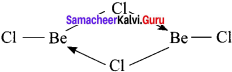2. BeCl2 (solid):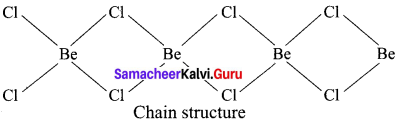Question 61.
Why is Li2CO3 decomposed at a lower temperature whereas Na2CO3 at higher temperature?
Li2CO3 is a covalent compound, whereas Na2CO3 is an ionic compound. Therefore, lattice energy of Na2CO3 is higher than that of Li2CO3. Thus, LiCO3 is decomposed at a lower temperature.

Question 62.
Alkali metals give colouration when heated in Bunsen flame. Give reason.
1. When the alkali metals salts moistened with concentrated hydrochloric acid are heated on a platinum wire in a flame, they show characteristic coloured flame.

• Lithium – Crimson red
• Sodium – Yellow
• Potassium – Lilac
• Rubidium – Reddish violet
• Caesium – Blue

2. The heat in the flame excites the valence electron to a higher energy level. When it drops back to its actual energy level, the excess energy is emitted as light whose wavelength is in the visible region produces colour.

Question 63.
How sodium metal reacts with –
1. ethanol
2. acetylene.
(i) Sodium metal reacts with ethanol to form sodium ethoxide and liberates H2 gas.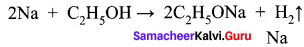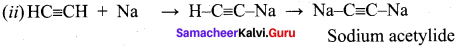Question 64.
Mention the uses of lithium and its compounds. .

• Lithium metal is used to make useful alloys. For e.g. with lead, lithium is used to make white metal bearings for motor engines, with aluminium to make aircraft parts and with magnesium to make armour plates.It is also used in thermonuclear reactions.
• Lithium is used to make electrochemical cells.
• Lithium carbonate is used in medicines.

Question 65.
What are the uses of sodium and its compounds?

• Sodium is used to make Na/Pb alloy needed to make Pb(Et)4 and Pb(Me)4. These organolead compounds were used as anti-knock additives to petrol in early days.
• Liquid sodium metal is used as a coolant in fast breeder nuclear reactors.

Question 66.
What are the uses of potassiunt and its compounds?

• Potassium has a vital role in biological system.
• Potassium chloride is used as a fertilizer.
• Potassium hydroxide is used in the manufacture of soft soap.
• Potassium hydroxide is also used as an excellent absorbent of carbon dioxide.

Question 67.
What is soda ash? How is it obtained?
Sodium carbonate decahydrate commonly known as washing soda Na2CO3. 10H2O. Upon heating, it looses the water of crystallization to form monohydrate. Above 373K, the monohydrate becomes completely anhydrous and changes to a white powder called soda ash.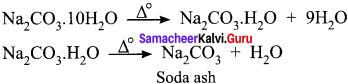Question 68.
Mention the uses of washing soda (or) sodium carbonate.

• Sodium carbonate is highly used in laundering.
• It is an important laboratory reagent used in the qualitative analysis and in volumetric analysis.
•  It is also used in water treatment to convert the hard water to soft water.
• It is used in the manufacture of glass, paper and paint.

Question 69.
How would you prepare pure sodium chloride from crude salt?
(a) Crude salt contains sodium sulphate, calcium sulphate, calcium chloride and magnesium chloride as impurities along with sodium chloride.
(b) Pure NaCl is obtained from crude salt by removal of insoluble impurities through filtration from the crude salt solution with minimum amount of water.
(c) Sodium chloride can be crystallized by passing HCl gas into this solution.
(d) Calcium and magnesium chloride being more soluble than NaCl, remain in solution.

Question 70.
Mention the uses of sodium chloride.

• It is used as a common salt (or) table salt for domestic purpose.
• It is used for the preparation of many inorganic compounds such as NaOH and Na2CO3.

Question 71.
What are the uses of sodium hydroxides?

• Sodium hydroxide is used as laboratory reagent.
• It is used in the purification of bauxite and petroleum refining.
• It is used in the textile industries for mercerising cotton fabrics.
• It is used in the manufacture of soap, paper, artificial silk and a number of chemicals.

Question 72.
Give reason why sodium bicarbonate is used in bakeries.
Sodium bicarbonate is called as baking soda. Because it decomposes on heating to generate bubbles of carbon dioxide, leaving holes in cakes or pastries and making them light and fluffy.

Question 73.
Write about the uses of sodium bicarbonate.
(a) NaHCO3 is used as an ingredient in baking.
(b) It is used as a mild antiseptic for skin infections.
(c) It is also used in fire extinguishers.

Question 74.
Explain the action of soda lime with –
1. SiO3
2. P4O10.
Quick lime mixed with soda gives solid soda lime. It combines with acidic oxides such as SiO2, ançi P4O10 to form calcium silicate and calcium phosphate, respectively.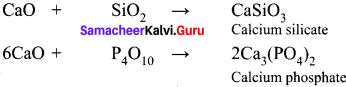Samacheer Kalvi 11th Chemistry Alkali and Alkaline Earth Metal 3-Mark Questions

Question 1.
Explain the periodic nature of ionization enthalpy in the alkali group.

1. Alkali metals have the lowest ionization enthalpy in each period.
2. Within the group, as we go down, the ionization enthalpies of alkali metals decreases due to the increase in atomic size.
3. In large atoms, the valence electrons are loosely held by the nucleus and arc easily lost, leading them to have low ionization enthalpy and acquiring stable noble gas configuration.
4. On moving down the group, the atomic size increases and the number of inner shells also increases, which in turn increases the magnitude of screening effect. So. the ionization enthalpies decreases down the group.

Question 2.
Explain the various periodic trends in the alkali metals down the group.
in alkali metals, as we move down the group

• reactivity increases
• screening effect increases
• tendency to form cation by loosing outer electron increases
• melting point and boiling point decreases

Question 3.
Explain about the anomalous behaviour of lithium among the alkali metals.

• Lithium is extremely small.
• It has great polarizing power.
• It has least electropositive character.
• In lithium, non-availability of d-orbitais is observed.

Question 4.
How alkali metals react with oxygen? Explain with equation.
1. All the alkali metals on exposure to air burn vigorously, forming oxides on the surface of the metals.
2. Lithium forms only monoxide, sodium forms the monoxide and peroxide and the other elements form monoxide, peroxide and superoxides.
4Li + O2 → 2Li2O (Lithium monoxide)
2 Na + O3 → Na2O2 (Sodium peroxide)
K + O3 → KO2 (Potassium superoxide)

Question 5.
How alkali metals react with liquid ammonia?

• Alkali metals dissolve in liquid ammonia to give deep blue solutions that are conducting in nature.
• This happens because the alkali metal atom readily loses the valence electron in ammonia solution.
• Both the cation and the electron combine with ammonia to form ammoniated cation and ammoniated electron. M + (x+v)NH3 → [M(NH3)x]+ + [e(NH3)y]

Question 6.
What is the reason behind the blue colouration of alkali metals with liquid ammonia?
M + (x + v)NH3 → [M(NH3)x]+ + [e(NH3)y]
The blue colour of the solution is due to the ammoniated electron which absorbs energy in the visible region of light and thus imparts blue colour to the solution. The solutions are paramagnetic and on standing slowly liberate hydrogen resulting in the formation of amide. In concentrated solution, the blue colour changes to bronze colour and become diamagnetic.

Question 7.
Explain how alkali metal oxide reacts with water?
Alkali metal oxides M2O, M2O2 and MO2 are easily hydrolyzed by water to form the hydroxides
according to the following reactions:
M2O + H2O → 2M+ + 2OH
M2O, + 2H2O → 2M+ + 2OH + H2O2
2MO2 + 2H2O → 2M+ + 2OH H2O2 + O2

Question 8.
Describe about the fire works of alkaline earth metals.

• Combined with the element of chlorine, barium sends up a green spark.
• Strontium chloride flashes red.
• Copper and chlorine compound makes a blue firework.
• Magnalium – A mixture of the alkaline earth metal magnesium and aluminium boosts all fire works colours, particularly makes the blue brighter.

Question 9.
Copper and chlorine compounds makes blue fire work Why?

• To produce colours, fire works experts hum the metal and chlorine together in a vapour, where the two elements are gases instead of solid.
• The burning excites the electron pushing them into a higher than normal energy level. As the electrons returns to their normal level, they release their extra energy as a colourful burst of light.
• True blue fireworks arc the hardest to make since the compound copper chloride breaks down in a hot flame.

Question 10.
IE1of alkaline earth metals are higher than that of alkali metals, but IE2 of alkaline earth metals are smaller than that of alkali metals. Give reason.

1. IE1 of alkaline earth metals > IE1 of alkali metals.
2. IE2 of alkaline earth metals < IE2 of alkali metals.
3. This occurs because in alkali metals the second electron is to be removed from a cation, which has already acquired a noble gas configuration.
4. In the case of alkaline earth metals, the second electron is to be removed from a monovalent cation, which still has one electron in the outermost shell.
5. Thus, the second electron can be removed more easily in the case of group 2 elements than in group I elements.

Question 11.
MgCl2 and CaCl2 are easily hydrated, while NaCl and KCl are not hydrated. Why?
Compounds of alkaline earth metals are more extensively hydrated than those of alkali metals, because the hydration enthalpies of alkaline earth metal ions are larger than those of alkali metal ions.
e.g., MgCl2 and CaCl2 exist as MgCl2.6H2O and CaCl2.6H2O, while NaCl and KCl do not form such hydrates.

Question 12.
What are the distinctive behaviour of beryllium?

• Beryllium is small in size.
• It has high polarizing power.
• Its electronegativity is relatively high.
• It has high ionization enthalpy.
• In valence shell, vacant d-orbitais are absent in beryllium.

Question 13.
Write about the important uses of calcium. Calcium is used

• As a reducing agent in the metallurgy of uranium, zirconium and thorium.
• As a de oxidizer, desulfurizer or decabonizer for various ferrous and non-ferrous alloys.
• in making of cements and mortars to be used in construction.
• As a getter in vacuum tubes.
• In dehydrating oils
• In fertilizers, concrete and Plaster of Paris.

Question 14.
Mention about the uses of barium. Barium is used

• In metallurgy, its compounds are used in pyrotechnics, petroleum mining and radiology.
• De oxidizer in copper refining.
• Its alloys with nickel which readily emits electrons hence used in electron tubes and in spark plug electrodes.
• As a scavenger to remove last traces of oxygen and other gases in television and other electronic tubes.
• An isotope of barium ‘33Ba., used as a source in the calibration of gamma ray detectors in nuclear chemistry.

Question 15.
Be(OH)2 is amphoteric in nature. Prove it.
Be(OH)2 is amphoteric in nature as it reacts with both acid and alkali.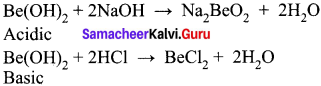Question 16.
Write a note about the structure of beryllium chloride.

• BeCl2 has a chain structure in the solid state.
• In the vapour phase BeCl2 tends to form a chioro-bridged dimer.
• At high temperatures of the order of 1200K it gives linear monomer.

Question 17.
Draw the structure of BeCl2 in different physical states.
1. In solid state:2. in vapour state: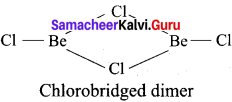3. in High temperature: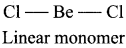Question 18.
Write about the suiphates of alkaline earth metals.

• The sulphates of the alkaline earth metals are all white solids and stable to heat.
• BeSO4 and MgSO4 are readily soluble in water; the solubility decreases from CaSO4 to BaSO4.
• The greater hydration enthalpies of Be2and Mg2 ions overcome the lattice enthalpy factor and therefore their suiphates are soluble in water.
Solubility order : BeSO4 < CaSO4 < BaSO4

Question 19.
What are the common physical and chemical features of alkali metals?
Physical properties of alkali metals :

• Alkali metals have low ionization enthalpies.
• Alkali metals are highly electropositive in nature.
• Alkali metals exhibit +1 oxidation states in their compounds.
• Alkali metals impart characteristic colours to the flame.

Chemical properties of alkali metals:

• Alkali metals are highly reactive in nature.
• Alkali metals hydroxides are highly basic in nature.
• Alkali metals dissolve in liquid ammonia to form blue and conducting solution.

Question 20.
Compare the alkali metals and alkaline earth metals with respect to –

1. ionization enthalpy
2. basicity of oxides and
3. solubility of hydroxides.

1. Ionization enthalpy:
Because of high nuclear charge the ionization enthalpy of alkaline earth metals are higher than those of the corresponding alkali metals.

2. Basicity of oxides:
Basicity of oxides of alkali metals are higher than that of alkaline earth metals.

3. Solubility of hydroxides:
Solubility of hydroxides of alkali metals are higher than that of alkaline earth metals. Alkali metals due to lower ionization enthalpy are more electropositive than the corresponding group 2 elements.

Question 21.
Why is Li2CO3 decomposed at a lower temperature, whereas Na2CO3 at higher temperature?
Li2CO3 is a covalent compound, whereas Na4CO3 is an ionic compound. Therefore, lattice energy of Na2CO3 is higher than that of Li2CO4. Thus, Li2CO3 is decomposed at a lower temperature.

Question 22.
What happens when

• Sodium metal is dropped in water?
• Sodium metal is heated in free supply of air?
• Sodium peroxide dissolves in water?

• 2Na + 2H2O —‘ 2NaOH + H2
• 2Na + O2 —+ Na2O2
• Na2O2+ 2H2O —‘ 2NaOH + H202

Question 23.
Write balanced equations for reactions between

1. Na2O2 and water
2. KO2and water
3. Na2O and CO2

1. Na2O2 + 2H2O → 2NaOH + H2O2
2. 2KO2 + 2H2O → 2KOH + O2 + H2O2
3. Na2O + CO2 → Na2CO3

Question 24.
How would you explain the following observations?

1. BeO is almost insoluble but BeSO4 is soluble ¡n water.
2. BaO is soluble but BaSO4 is insõluble in water.
3. LiI is more soluble than KI in ethanol.

1. Lattice energy of BeO is comparatively higher than the hydration energy. Therefore, it is almost insoluble in water. Whereas BeSO4 is ionic in nature and its hydration energy dominates the lattice energy.
2. Both BaO and BaSO4 are ionic compounds but the hydration energy of BaO is higher than the lattice energy, therefore it is soluble in water.
3. Since the size of Li+ ion is very small in comparison to K+ ion, it polarises the electronb cloud of I ion to a great extent. Thus LiI dissolves in ethanol more easily than the KI.

Question 25.
Explain the following:

1. Why Cs is considered as the most electropositive element?
2. Lithium cannot be used in making photoelectric cells.
3. Lithium does not form alums.

1. Due to its lowest ionization energy, Cs is considered as the most electropositive element.
2. Lithium cannot be used in making photoelectric cells because out of all the alkali metals, it has highest ionization energy and thus cannot emit electrons when exposed to light.
3. Due to small size, lithium does not form alums.

Question 26.
Give the important uses of the following compounds.
1. NaHCO3
2. NaOH
1. Uses of NaHCO3

• It is used in fire extinguisher.
• It is used as mild antiseptic for skin infections.
• It is used as antacid.

2. Uses of NaOH

• It is used in soap industry
• It is used as reagent in laboratory
• It is used in absorbing poisonous gases.

Question 27.
The hydroxides and carbonates of sodium and potassium are easily soluble in water, while the corresponding salts of magnesium and calcium are sparingly soluble in water. Explain.
All the compounds are crystalline solids and their solubility in water is guided by both lattice enthalpy and hydration enthalpy. The magnitude of lattice enthalpy is quite small in case of sodium and potassium compounds, hence they are readily dissolved in water, when compared to magnesium and calcium compounds.

However, in case of corresponding magnesium and calcium compounds, the cations have smaller sizes and more magnitude of positive charge. This means that their lattice enthalpies are more, when compared to the sodium and potassium compounds. Therefore, the hydroxi des and carbonates of these metals are only sparingly soluble in water.

Question 28.
Why is LiF almost insoluble in water, whereas LiCl soluble not only in water but also In acetone?
The low solubility of LiF in water is due to its very high lattice enthalpy (F ion is very small in size). On the other hand, in lithium chloride (LiCl) the lattice enthalpy is comparatively very small. This means that the magnitude of hydration enthalpy is quite large. Therefore lithium chloride dissolves in water. It is also soluble in acetone due to dipolar attraction (Acetone is polar in nature).

Question 29.
Which out of the following can be used to store an alkali metal?
1. H2O
2. C2H5 OH
3. benzene
3. Benzene can be used to store an alkali metal, because other substances react with alkali metal as below:
Na + H2O → NaOH + ½H2
Na + C2H5COH → C2H5ONa + ½H2

Samacheer Kalvi 11th Chemistry Alkali and Alkaline Earth Metal 5 – Mark Questions

Question 1.
Explain in what respects lithium ¡s different from other metals of the same group.
lithium:

• Very hard.
• High melting and boiling point.
• Least reactive.
• Reacts with nitrogen to get Li3N.
• Reacts with bromine slowly.
• Burnt in air gives monoxide only.
• Compounds are partially soluble in water.
• Lithium nitrate decomposes to fòrm an oxide.
• Extremely small in size.
• Li+ has greater polarizing power.

Other elements of the family:

• Very Soft.
Low melting and boiling point.
• More reactive.
• No reaction.
• Reacts violently.
• Burnt in air gives peroxides also, apart from monoxides. K, Rb and Cs gave super oxides.
• Highly soluble in water.
• Other metals on heating gives nitrite.
• Comparatively large in size.
• Other M+ ions have comparatively larger polarizing power.

Question 2.
Describe about the biological importance of sodium and potassium.
1. Monovalent sodium and potassium ions are found in large proportions in biological fluids.
2. These ions perform important biological functions such as maintenance of ion balance and nerve impulse conduction.
3. Sodium – Potassium play an important role in transmitting nerve signals.
4. Atypical 70 kg man has 90g of Na and 170 g of K.

5. Sodium ions are found on the outside of cells, being located in blood plasma and in the interstitial fluid which surrounds the cells. These ions participate in the transmission of nerve signals, in regulating the flow of water across cell membranes and in the transport of sugars and amino acids into cells.

6. Potassium ions are the most abundant cations within cell fluids, where they activate many enzymes, participate in the oxidation of glucose to produce ATP and with sodium, are responsible for the transmission of nerve signals.

Question 3.
Compare the properties of beryllium with the other elements in the same group.

Beryllium:

• Forms covalent compounds.
• High melting and boiling point.
• Does not react with water even at elevated temperature.
• Does not combine directly with hydrogen.
• Halides are covalent.
• Hydroxides and oxides of beryllium are amphoteric in nature.
• It is not readily attacked by acids because of the presence of an oxide film.
• Beryllium carbide evolves methane withwater.
• Salts of Be are extensively hydrolyzed.
• It has no vacant ‘d’ orbitals in the outermost shell.

Other elements of the family:

• Forms ionic compounds.
• Lower melting and boiling point.
• React with water.
• Combine directly with hydrogen.
• Halides are ionic or electrovalent.
• Hydroxides and oxides are basic in nature.
• Other carbides evolves acetylene with water.
• Hydrolyzed.
• They have vacant ‘d’ orbitais in the outermost shell.

Question 4.
List out the uses of magnesium.
Magnesium is used:

• in the removal of sulphur from iron and steel.
• in the refining of titanium in the “Kroll” process.
• as photoengrave plates in printing industry.
• Magnesium alloys are used in airplane and missile construction.
• Mg ribbon is used in synthesis of Grignard reagent in organic synthesis.
• It alloys with aluminium to improve its mechanical, fabrication and welding property.
• as a desiccant.
• as sacrificial anode in controlling galvanic corrosion.
• Magnesium is the co-factor of all enzymes that utilize ATP in phosphate transfer and energy release.

Question 5.
Distinguish between alkali metals and alkaline earth metals.
Alkali Metals:

• Alkali metals are soft.
• They have a single electron in the valence shell and their electronic configuration is [noble gas] ns1.
• They have low melting points.
• Hydroxides are strongly basic.
• Carbonates do not decompose.
• Carbonates do not decompose.
• Nitrates give corresponding nitrites and oxygen as products.
• They show +1 oxidation states.
• Their carbonates are soluble in water except Li2CO3.
• Except Li, alkali metals do not form complex compounds.

Alkaline earth metals:

• Alkaline earth metals are hard.
• They have two electrons in the valence shell and their electronic configuration is [noble gas] ns2.
• They have relatively high melting points.
• Hydroxides are less basic.
• Carbonates decompose to form oxide, when heated to high temperatures.
• Carbonates decompose to form oxide, when heated to high temperatures.
• Nitrates give corresponding oxides, nitrogen dioxide and oxygen as products.
• They show +2 oxidation states.
• Their carbonates are insoluble in water.
• They can form complex compounds.

Question 6.
State as to why
(a) Alkali metals show only +1 oxidation state.
(b) Na and K impart colour to the flame but Mg does not.
(c) Lithium on being heated in air mainly forms the monoxide and not the peroxide.
(d) Li is the best reducing agent in aqueous solution.
(a) Alkali metals have low ionization enthalpies. They have a strong tendency to lose 1 electron to form unipositive ions. Thus they show an oxidation state of+1 and are strongly electropositive.

(b) Valence electrons of alkali metals like Na and K easily absorb energy from the flame and are excited to higher energy levels. When these electrons return to the ground state, the energy is emitted in the form of light. Magnesium atom has small size so electrons are ’ strongly bound to the nucleus. Thus they need large amount of energy for excitation of electrons to higher energy levels, which is not possible in Bunsen flame.

(c) Due to the small size of Li+ it has a strong positive field, which attracts the negative charge so strongly that it does not permit the oxide ion (O2-) to combine with another oxygen atom to form peroxide ion.

(d) Among alkali metals, lithium has the most negative electrode potential (Ee = -3.04 V), so it is the strongest reducing agent in the aqueous solution.

Question 7.
An alkali metal (A) belongs to period number II and group number I react with oxygen to form (B). (A) reacts with water to form (C) with liberation of hydrogen compound (D). Identify A, B, C and D.
1. An alkali metal (A) belongs to period number II and group number I is lithium.
2. Lithium reacts with oxygen to form simple oxide lithium oxide (B).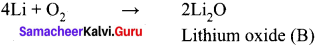3. Lithium reacts with water to form lithium hydroxide with liberation of hydrogen.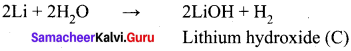4. Lithium directly react with carbon to form an ionic compound lithium carbide.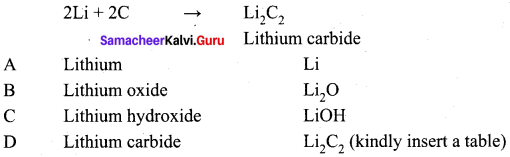Question 8.
Describe Solvay process (or) how is washing soda (or) sodium carbonate prepared in industries?
(i) Solvay process – in this process ammonia is converted to ammonium carbonate, which is then converted to ammonium bicarbonate by passing excess carbon dioxide in sodium chloride solution saturated with ammonia.
(ii)The ammonium bicarbonate formed reacts with sodium chloride to give sodium bicarbonate.
As sodium bicarbonate has poor solubility, it gets precipitated.
(iii)The sodium bicarbonate is isolated and is heated to give sodium carbonate.
(iv)The equations involved in this process is as below: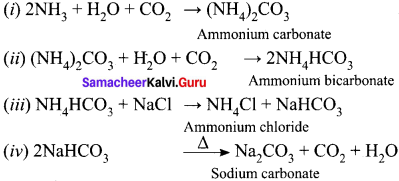Question 9.
Describe the method of electrolysis of brine solution? (or) how is sodium hydroxide prepared commercially?